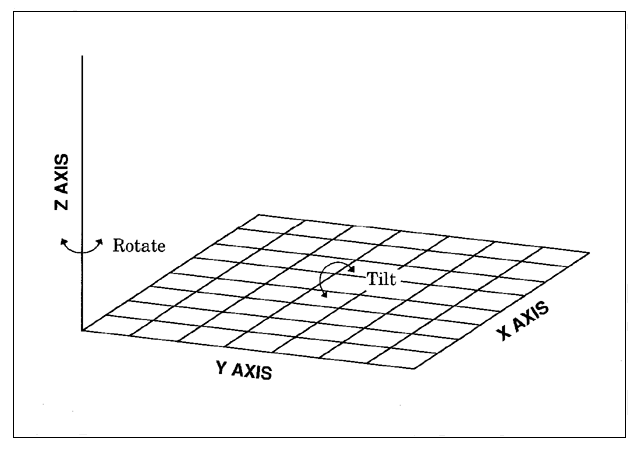# Rotating and Tilting the Plot

For both surface plots and scatter plots, you can rotate the X-Y plane around the Z axis, or tilt the X-Y plane toward you. When you rotate a plot, you can view data from any angle around the three-dimensional graph. Rotating a plot is useful for bringing into view data points that might be obscured by other data points. Tilting a plot enables you to accentuate the location of data points.
The following diagram illustrates how the TILT= option, and the ROTATE= option change the viewing angles of a plot.
Rotating and Tilting a PlotNote: Certain combinations of the TILT= option, and the ROTATE= option can cause the tick mark values to overlap.